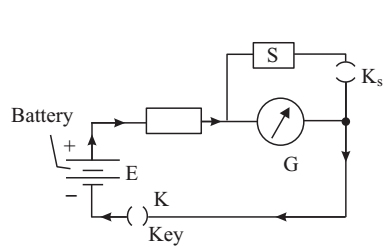# Internal Resistance of Galvanometer by Half Deflection Experiment

Experiment: To determine the internal resistance of a galvanometer by half deflection method, and to convert it into a volt meter of a given range, say (0-3 V), and verify it.

Material Required

A battery, a galvanometer (pointer type), a voltmeter of suitable range, 25 ohm - 3 A rheostat, 5000 ohm resistance box, 100 ohm resistance box, two one-way keys, D.C.C. copper wire for making connections and sand paper.

Whenever you record a deflection on the scale of a meter, there is always an uncertainty of ± 0.5 division in a reading, which results in an error of 1 division in the observed deflection. Therefore, for accurate results from the observations on deflection instruments, as far as possible, you should choose a meter of such range that the deflections produced by the current or potential difference to be measured is 70% or more on the scale.

In this experiment you will use a Galvanometer and a D.C. voltmeter. The letters G, V respectively stand for Galvanometer and Voltmeter. The underline below the letters means that these instruments are meant for D.C. only. For determination of galvanometer resistance G the circuit is connected.Let the current flowing through the Galvanometer be I and corresponding deflection in it be θ. Then, connect the resistance S in parallel with galvanometer and adjust its value so that the deflection in galvanometer becomes half, i.e. θ/2. Now, the current flowing through the galvanometer is I/2 and remaining I/2 is by-passed by the resistance S connected across G. Because the current divides equally between G and S, therefore,

G = S

The resistance S connected across a part of circuit to reduce current in that part only, is called the shunt. Another important constant of a Galvanometer is Ig, the full scale deflection current. Ig is that much current which deflects the Galvanometer pointer from 0 to maximum deflection on its scale.

To convert a Galvanometer into a voltmeter of desired range say (0-V volts), a suitable high resistance Rs is connected in series with the Galvanometer.

The value of resistance Rs is such that if a PD of V volt is connected across the combination of Rs and G, a current I flows through it and produces a full scale deflection in the galvanometer. Applying Ohm’s law,

(Rs + G) = V/Ig

Rs = V/Ig – G

### How To Perform Experiment

A. To determine the G and I of galvanometer

1. Set the needle to zero in the galvanometer and voltmeter.

2. Note the least count of voltmeter.

3. Measure the emf of lead accumulator with voltmeter and record at the top of observation table.

4. Place the equipment and connect them with pieces of DCC wire whose ends have been properly cleaned with sand paper. Take out 5 k ohm key from the resistance box R and insert key K. Adjust R so that the deflection in G is more than 20 (70% of number of divisions in the galvanometer scale) and divisible by 2, say 22.

5. Now insert the shunt key Ks as well. Deflection in galvanometer will fall. Adjust the value of S by taking out various keys from it till the deflection in galvanometer is θ/2, i.e. 11 in this set. Record the values of R, θ, and S in observation table. Now repeat the process with different values of R to get deflections 24, 26, etc. and reducing the deflection from θ to θ/2 in each case.

You will see that the value of S will come out to be same in each case. Record R, S and θ in table and calculate Ig.

B. Conversion of a galvanometer into a voltmeter of range V

6. After having determined the internal resistance G and full scale deflection current Ig of the given galvanometer, calculate the series resistance Rs. Connect this resistance in series with the galvanometer. The galvanometer will become a voltmeter of range V.

To check the correctness of conversion of the Galvanometer, compare it with a standard voltmeter.

7. Keep the moving terminal C of the rheostat near terminal A and insert the key K. Now move the terminal C towards terminal B and note the readings of standard voltmeter and converted voltmeter in steps of 0.5 V. Record these readings. Find the difference between the readings of two meters. If it is zero then the conversion is O.K.

### Sources of Error

1. The ends of connecting wires may not be clean thus causing contact resistance.
2. The keys of resistance box may not be tight enough and may have contaminated surface if not cleaned by a cleaning liquid.
3. If deflection with key Ks open, is less then 70% of full scale, percentage error of the experiment shall be quite large.
4. There may be parallax error in observing deflection.
5. In half deflection method the total current is assumed constant but in fact it increases when shunt is connected.